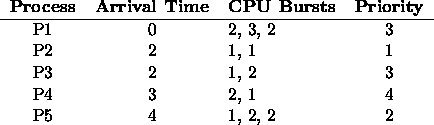# Homework 2

CS42

50 pts., due Oct. 4

1. (10 pts.) What two advantages do threads have over multiple processes? What major disadvantage do they have? Suggest one application, not discussed in class, that would benefit from the use of threads, and one that would not.

2. (15 pts.) Consider a system in which mailboxes are used for IPC. A kernel-level disk process is associated with the single disk drive in the system and it handles read/write requests from user-level processes. A system library provides fread() and fwrite() functions for user-level processes to access a disk. The functions are defined:
1. int fread(int sector, char *buff) --- Read data sector sector from the disk into buff. Block until the operation completes, returning 1 on success and 0 on failure.
2. int fwrite(int sector, char *data) --- Write data from data to disk sector sector, blocking until the operation completes. Return 1 on success and 0 on failure.

Write pseudo-code for the disk process and the two system level library functions. Clearly indicate the content of mailbox messages.

3. (15 pts.) Consider the following set of processes:Draw four Gantt charts illustrating the execution of these processes using FCFS (non-
preemptive), preemptive SJF, non-preemptive priority (a smaller priority number implies a higher priority), and RR (quantum = 1).

Also, for each scheduling algorithm, answer the following:

1. What is the turnaround time of each process?

2. What is the waiting time of each process?

Which algorithm results in the smallest average waiting time?

4. (10 pts.) The first known correct software solution to the CS problem was proposed by Dekker:
```int flag = { FALSE, FALSE }   /* flag[i] indicates that Pi wants to */
/*  enter its critical section */
int turn = 0;                    /* turn indicates which process has */
/*  priority in entering its critical */
/*  section

flag[i] = TRUE;
while (flag[1 - i])
if (turn == 1 - i) {
flag[i] = FALSE;
while (turn == 1 - i)
;
flag[i] = TRUE;
}
CSi;
turn = 1 - i;
flag[i] = FALSE;
```
Prove that the algorithm satisfies the three requirements for a solution to the CS problem.

Thomas P. Kelliher
Thu Sep 26 22:29:35 EDT 1996
Tom Kelliher